Journal of Photonic Materials and Technology
Volume 2, Issue 1, July 2016, Pages: 1-5

Enhancement of the Electromagnetic Waves Intensity by Scattering on Multiple Infinite Cylinders

Arnold Abramov1, Chunlin Ji1, Ruopeng Liu1, Alexander Kostikov2

1Kuang-Chi Institute of Advance Technology, Shenzhen, China

2Donbass State Engineering Academy, Kramatorsk, Ukraine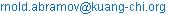(A. Abramov)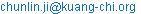(Chunlin Ji)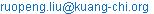(Ruopeng Liu)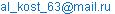(A. Kostikov)

Arnold Abramov, Chunlin Ji, Ruopeng Liu, Alexander Kostikov. Enhancement of the Electromagnetic Waves Intensity by Scattering on Multiple Infinite Cylinders. Journal of Photonic Materials and Technology. Vol. 2, No. 1, 2016, pp. 1-5. doi: 10.11648/j.jmpt.20160201.11

Received: May 6, 2016; Accepted: June 16, 2016; Published: July 5, 2016

Abstract: We report the effect of multiple cylinders scattering of electromagnetic plane-wave on formation of high field intensity areas. Our model consists of three cylinders. One of them was basic and the possibility of the existence of high intensity field which can form in its vicinity has been studied. Two values of the radius for basic cylinder were considered: a resonant radius when the so-called whispering gallery modes formed, and non-resonant. It was found that a focal length of photonic nano jets generated at the shadow-side surface of basic cylinder increased (up to two times) compared to the case of a single cylinder. For whispering gallery modes the field intensity also increased up to two times.

Keywords: Nanojet, Diffraction, Cylinder, Resonant, Wavelength

Contents

1. Introduction

Sub wavelength imaging can be defined as the ability to see details of the object having one or more dimensions smaller than the length of the wave with which the object interacts; while usually the minimum length scale on which a beam of light can operate is equal to half of its wavelength. The sub wavelength imaging has potential applications in biology, photo- and nanolithography, spectroscopy.

Sub wavelength imaging can be also considered as overcoming of so called diffraction limit. The latter appears because a general expansion of an EM field emanating from a source consists of both propagating waves and evanescent waves. Evanescent waves decay in the direction of propagation and are always lost, but because they corresponds to a high angular frequency components of the wave, which contain information about the small scale features of the object being imaged, this lead to the diffraction limit for focusing to an image. Different kind of optical microscopy technique has been developed [1-5] to make possible spectroscopic measurements having a spatial resolution below the diffraction limit. Principally new way to overcome the diffraction limit was discovered in Ref.  where the authors found and studied a narrow, high-intensity beam of light generated at the shadow-side surface of plane-wave-illuminated dielectric cylinders. At that the beam waists is much smaller than the wavelength and sub wavelength imaging becomes possible. Thereby one can see details of an object below the wavelength of visible light. Photonic nanojet could be formed also by spheres , multilayers cylinders , discs . The influence of incident light polarization was investigated in . It was shown that radially polarized light formed photonic nanojet with effective focal volume 10 times smaller than the circularly polarized light. Dielectric sphere with properly graded refractive index was used in  to increase the length of photonic nanojet. A nanojet generated by dielectric micro cylinders with different metallic coatings has been analyzed numerically in . Adding truncated concentric rings to the cylinder increases the depth of focus to 1.18 of the wavelength . Formation and transport of photonic nano jets for multiple cylinder scattering have been calculated in Ref. .

While photonic nanojet was recently discovered thanks to new computational capabilities, an existence of a region with high field intensity inside the cylinder due to the presence of whispering gallery modes (WGMs) is a well known and established fact [15, 16]. In Ref.  it was shown that WGM with the number 30 forms a focal spot outside the cylinder with width of 0.15λ (λ - wavelength) and maximum intensity to 1500 times greater than the intensity of incident light.

In the present paper we study how positions of the cylinders affect the formation of high field intensity areas. Our model consists of three cylinders. One of them is basic, in which and around which we consider the formation of high intensity field areas. The two other has assistive function. Their positions vary relative to the basic cylinder, with the aim to find the positions optimizing the characteristics of the scattered field.

2. Modeling

Our model as well as distances and notations presented on Fig. 1. Plane wave falls from the left side on the group of three cylinders. The direction of wave propagation is along the axis of symmetry of the cylinders which selected as the x-axis.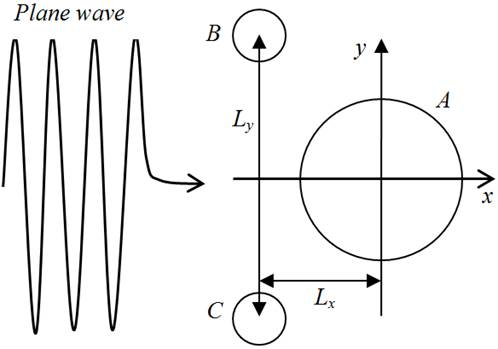Fig. 1. Geometry to simulate scattering of plane wave by multiple cylinder.

The origin of coordinates corresponds to the center of the main cylinder A.

The propagation and scattering of the electromagnetic plane wave was studied using MATLAB toolbox developed in Ref. . The original formulation of the problem, as well as a system of wave equations for scattered and transmitted wave field is presented in . The conditions of the equality on the boundary for the fields and their normal derivatives inside and outside the cylinder where used as boundary condition. Solution of the problem was found as Fourier series expansion. The accuracy of final results was controlled by number of terms in the expansion.

TE-polarized plane wave (λ = 532 nm) was used as the incident wave. The mesh grid size in space was equal to 0.04 μm (0.075 of wavelength).

Whispering gallery modes can be treated as modes confined in dielectric cylinder due to the total internal or Bragg reflection. In order to calculate corresponding frequency we consider the expression for the electric field with TE polarization inside the cylinder in the form of an expansion in series with Bessel functions: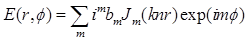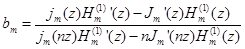where z=kR; wave vector k=2π/λ, Jm(z)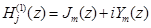. Using well known properties of cylindrical functions  this expression can be rewritten asFor each value of m there is a value of R at which the contribution of the m-th mode is dominant. As example, we present on Fig. 2 the dependences of bm(R/λ) for m=5, 10, 15, 20, 25. For the small values of z Re(W) >> Im(W) and maximum of bm is in the vicinity of zm determined as minimal root of the equation Re(W)=0. As m increases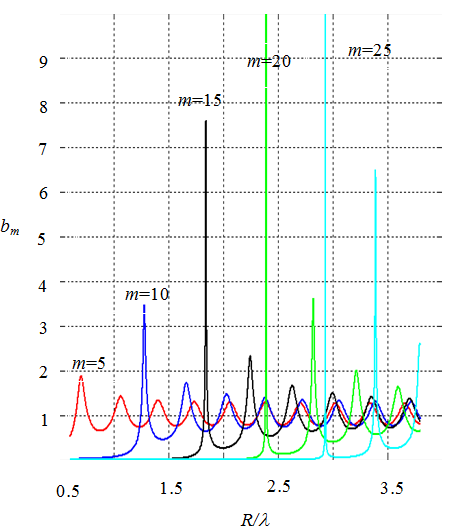Fig. 2. Dependence of bm versus R/λ for different m. The maximums for m=20 and m=25 are truncated and are of 18.86 and 50.59 respectively.

position of maximum bm(z) corresponds to zm. Then let us rewrite W performing the linear expansion of W near the value of zm: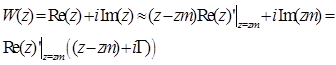Here, the expression for peak width is introduced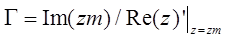The calculated width for different m is shown in Fig. 3.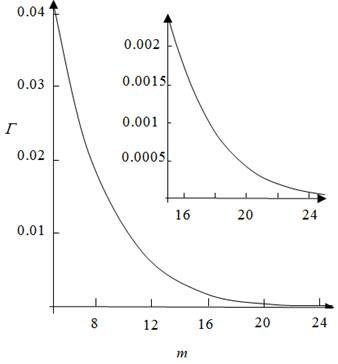Fig. 3. Half width of maximum bm for different m. inset: same, but more detail for range m=16..24.

The half-width of the peak decreases as m increase, and reaches »0.000064 for m=25. Thus choosing the value of radius R corresponding to the maximum of bm(R/λ) with the largest m, we restrict the possible error in the selection of the radius for cylinder, because the deviation in the radius more than Γ leads to a sharp decrease in the contribution of corresponding mode. With this assuming we chose the radius of the cylinder corresponding to the maximum of bm with m=15. The value of Γ in this case is equal to 0.0024 (R/λ).

Consider now our model presented on Fig. 1. Cylinder’s A radius RA=1.84394λ provides the maximum of coefficient bm for m=15. The radii of the cylinders B and C are of 0.5RA. Changing the coordinates of centers of the cylinders B and C (i.e. the distances Lx and Ly), we tried to find the positions corresponding to the maximum field intensity around cylinder A. We found it for the values Lx= -0.9 μm, Ly=2.5 μm. The comparative picture for the case of single cylinder A and our model is shown in Fig. 4. It is seen that the maximum value of the field in our model is greater approximately in 1.5 times (respectively, intensity – in 2.25 times).

The location of the focus near the surface means that it is formed mainly by surface wave, which is in this case a standing surface wave formed by the interference of two same WGMs, "running" toward each other along the cylinder surface in counterclockwise and clockwise directions.

In general case, the focus on the optical axis near the surface of the cylinder is formed by the interference of two waves: the wave passing through the cylinder and focused by the cylinder, and surface wave, which is a WGM. The contribution of these two waves in the focus is different and depends on the radius of the cylinder. The total energy of a WGM is determined by two processes: the replenishment of energy by wave impinging on a cylinder and power consumption due to the "leakage" of WGM from the cylinder. Leakage is proportional to stored energy and is inversely proportional to the radius of the cylinder. Therefore, for relatively large radii of the cylinder the contribution of the WGM intensity in the focus is much larger than the contribution of the intensity of the focused wave.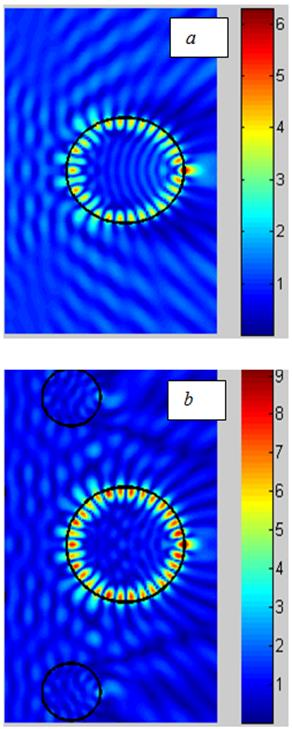Fig. 4. Intensity distribution of electromagnetic wave scattered by one (a) and three (b) dielectric cylinders. Larger cylinder have resonant radius RA=1.84394 λ.

Also as indicated in , as the resonant radius increase WGM extends along a less curved surface, and therefore, energy flows out of the cylinder less. Thus, the energy of the WGM stored within the cylinder increases with its radius. This energy is distributed between the petals of the WGM, one of which forms the focus.

However, there is also a spatial separation of the intensity maxima of these waves along the axis: WGM is formed near the surface of the cylinder, but inside the cylinder. On the contrary, passing through the cylinder wave focuses near the cylinder but outside it. With the increase of the radius of the cylinder "male" focus formed further away from the surface of the cylinder, increases in size and decreases in intensity. And petal of WGM which gives contribution to the focus, is located within the cylinder, and does not extend beyond the surface more than the wavelength.

The case of non-resonant radius is an interesting and important by next reasons: it is not necessary to match the radius of the cylinder, especially with high precision; it is possible to form a photonic nanojet outside of the cylinder. First, we obtained a picture for one cylinder of radius RA=2λ. It demonstrates the formed nanojet. Next we used the additional cylinders B and C with radii RB = RC = 0.5 RA. Changing the position of the coordinates of centers of cylinders B and C (i.e. the distances Lx and Ly), we tried to find a positions corresponding to the maximum extension of the photonic jet in the region x>RA. The length of nanojet Lext was calculated as the distance along the x axis from the surface of the cylinder to the point of half intensity.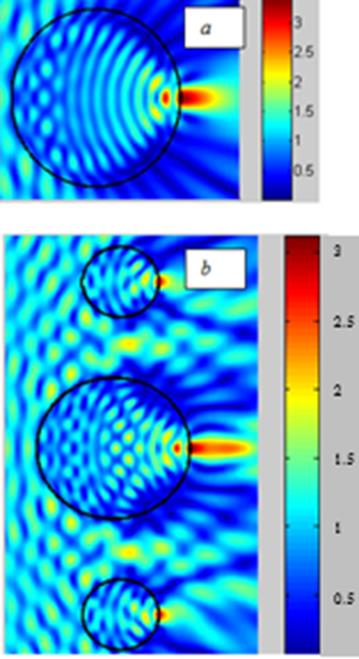Fig. 5. Intensity distribution of electromagnetic wave scattered by one (a) and three (b) dielectric cylinders. Larger cylinder have resonant radius RA=2 λ.

The comparative picture for the case of one cylinder and of our model when Lx =1, Ly =2 is shown in Fig. 5. It is seen that the extension of the nanojet in our model is greater at about 2 times. Fig. 6 shows the dependence of length of nanojet Lext and distance Ly versus the position of the cylinders B and C along the x axis. At that the Ly corresponds to the calculated Lext at the same position on the x axis (Lx value).

Since the contribution of the scattered field in nanojet is proportional to the exp (ikD), D - distance between centers of cylinders A and B or C, the values of the jet will be close for positions with difference order of λ. For large values of Lx (on the left side of Fig. 1) larger values of the Lext correspond to the remote positions of cylinders B and C along y axis. As cylinders B and C approached (reduction Lx) to the center then larger values of Lext meet positions more close to the x axis. It is seen by the sharp bending in Fig. 6 (b) at x= ̵ 0.1). Obviously, with further shift of cylinders B and C away the center the influence of the cylinders on the Lext will be offset, and is not of interest for the purposes of our work.

5. Conclusion

In conclusion, we have simulated scattering of plane wave by group of three cylinders. One of them was basic and the possibility of the existence of high intensity field in its vicinity has been studied. The radii of the cylinders A and B were chosen as RB=RC=0.5RA. Two values of the radius for cylinder A were considered: a resonant radius when the so-called WGMs can be formed, and non-resonant. The presence of cylinders B and C lead to increase of absolute value of the field in 1.5 times (as intensity maximum - in 2.25 times) for the resonant case. For the nonresonant case it was found that significant increase of nanojet length, as compared to the case of a single cylinder, occur if the centers of the cylinders are located near a plane perpendicular to the direction of the incident wave.

The present work demonstrates the ability to optimize the areas of high intensity field by multiple cylinders scattering, as well as further potential of this optimization by increasing the numbers of cylinder, their position, size, and physical characteristics.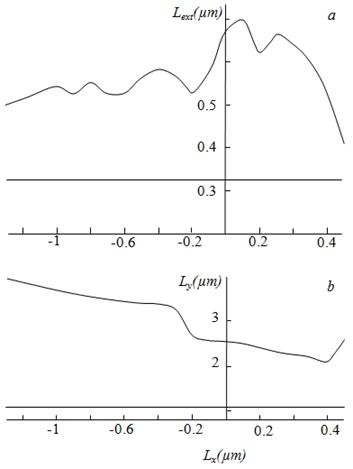Fig. 6. Extension of nanojet Lext (a) and distance Ly between cylinders B and C (b) versus position of cylinders B and C along x axis. Horizontal line: (a) – value of Lext for cylinder A with R=2λ; (b) – Ly=RA.

Acknowledgments

The work was supported by Guangdong Innovative Research Team Program (No. 2009010005), Shenzhen Innovative R&D Team Program (Peacock Plan) (No. KQE201106020031A), Shenzhen Key Laboratory of Optical and Terahertz Meta-RF (No. CXB201109210101A).

References

1. B. Dunn, "Near-field scanning optical microscopy," Chem. Rev. 99, 2891-2928 (1999).
2. S. M. Mansfield and G. S. Kino, "Solid immersion microscope," Appl. Phys. Lett. 57, 2615-2616 (1990).
3. L. Novotny, E. J. Sanchez, and X. S. Xie, "Near-field optical imaging using metal tips illuminated by highorder Hermite-Gaussian beams," Ultramicroscopy 71, 21-29 (1998).
4. B. B. Goldberg, S. B. Ippolito, L. Novotny, Z. Liu, and M. S. Ünlü, "Immersion lens microscopy of photonic nanostructures and quantum dots," IEEE J. Sel. Top. Quant. Elect. 8, 1051-1059 (2002).
5. J. B. Pendry, (2000). "Negative Refraction Makes a Perfect Lens" (PDF). Physical Review Letters 85 (18): 3966–9.
6. Z. Chen, A. Taflove, and V. Backman, "Photonic nanojet enhancement of backscattering of light by nanoparticles: a potential novel visible-light ultramicroscopy technique," Opt. Express 12(7), 1214–1220 (2004).
7. X. Li, Z. Chen, A. Taflove, and V. Backman, "Optical analysis of nanoparticles via enhanced backscattering facilitated by 3-D photonic nanojets," Optics Express, vol. 13, no. 2, pp. 526–533, 2005.
8. V. V. Kotlyar and M. A. Lichmanov, "Analysis of electromagnetic wave diffraction on an infinite circular cylinder with several layers of homogeneity," Computer Optics, vol. 24, pp. 26–32, 2002 (Russian).
9. D. McCloskey, J. J. Wang, and J. F. Donegan, "Low divergence photonic nanojets from Si3N4 microdisks," Optics Express, vol. 20, pp. 128–140, 2012.
10. Y. Liu, B. Wang, and Z. Ding, "Influence of incident light polarization on photonic nanojet," Chinese Optics Letters, vol. 9, no. 7, Article ID 072901, 2011.
11. S.-C. Kong, A. Taflove, and V. Backman, "Quasi one-dimensional light beam generated by a graded-index microsphere," Optics Express, vol. 17, no. 5, pp. 3722–3731, 2009.
12. C.-Y. Liu, Photonic nanojet enhancement of dielectric microcylinders with metallic coating. J. of Optoelectr. and Adv. Mater., 15, No.3 - 4, p. 150–154, 2013.
13. S. Stafeev and V. Kotlyar, Elongated Photonic Nanojet from Truncated Cylindrical Zone Plate, Journal of Atomic, Molecular, and Optical Physics, 2012, 1-3 (2012).
14. J. Schaefer, S.-C.Lee, A.Kienle, Calculation of the near fields for the scattering of electromagnetic waves by multiple infinite cylinders at perpendicular incidence, Journal of Quantitative Spectroscopy & Radiative Transfer 113, 2113–2123 (2012).
15. J. A. Stratton, Electromagnetic Theory (McGraw-Hill, New York, 1941).
16. S. Deng, W. Cai, V.Astratov, Numerical study of light propagation via whispering gallery modes in microcylinder coupled resonator optical waveguides, Opt. Expr., 12, No. 26, 6468-6480, 2004.
17. V.V. Kotlyar, D.A. Kozlov, A.A. Kovalev, Calculation of the resonant radius of a dielectric cylinder under illumination by a plane TE-wave, Computer Optics, vol. 39,No.2, pp. 163-171, 2015 (Russian).
18. B. Thierry, X. Antoine, C. Chniti and H. Alzubaidi.μ-diff: an open-source Matlab toolbox for computing multiple scattering problems by disks arXiv:1409.8186v1 [cs.MS] 29 Sep 2014
19. M. Abramovits and I. Stigan, Handbook on Special Functions [in Russian], Nauka, Moscow, 1979.

 Contents 1. 2. 3. 4. 5.
Article ToolsAbstractPDF(995K)